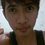# Multiplication Worksheets

Multiplication worksheets contain exclusive pages for selected topics such as multiplication facts, lattice multiplication, tables and charts, multiplication properties, in-out boxes and multiplication word problems. It also covers multipling numbers from single-digit to 4-digit numbers, identifying missing factor, multiplication using repeated addition, balancing equation and more.

Multiplication Facts Worksheets

Basic multiplication facts help kids to practice multiplication tables from 0 to 9. Single-digit multiplication worksheets included.

Multiplication Tables and Charts

Free printable tables and charts available to develop your skills in multiplication. Colorful one page tables included to paste it in the study room.

In and Out Boxes

Enter the input or output of the tables following the rule. This section helps the student to practice both multiplication and division.

Lattice Multiplication Worksheets

Multiply the numbers using the technique called lattice method. Teacher templates included to create their own worksheets.

Multiplication Properties

Multiplication properties worksheets include commutative, associative, distributive, identity and inverse properties.

Multiplication Word Problems

Practical applications easily understood with the help of these multiplication word problems. Word problems for larger digits included.

Multiplication Worksheets Simple Multiplication Practice

Basic multiplication worksheets include multiplying 2-digit and 3-digit numbers with single-digit numbers. Both column and horizontal multiplication included. The worksheets are suitable for grade 2 and up.

2-digit x 1-digit

Column Multiplication:

Column Multiplication 1

Column Multiplication 2

Column Multiplication 3

Horizontal Multiplication:

Horizontal Multiplication 1

Horizontal Multiplication 2

Horizontal Multiplication 3

3-digit x 1-digit

Printable multiplication worksheets include 3-digit and single-digit numbers.

Column Multiplication:

Printable Multiplication: Column 1

Printable Multiplication: Column 2

Printable Multiplication: Column 3

Horizontal Multiplication:

Printable Multiplication: Horizontal 1

Printable Multiplication: Horizontal 2

Printable Multiplication: Horizontal 3 Multiplication Practice: Moderate Level

The worksheets in this section are suitable for grade 4 and up. It includes multiplication of 2, 3, and 4 figure numbers with 2 figure numbers. Use long multiplication method to solve the worksheets.

2-digit x 2-Digit

Two by two digit multiplication worksheets:

2 Digit Multiplication 1

2 Digit Multiplication 2

2 Digit Multiplication 3

3-digit x 2-digit

Three by two digit multiplication worksheets:

3 and 2: Long Multiplication 1

3 and 2: Long Multiplication 2

3 and 2: Long Multiplication 3

4-digit x 2-digit

Four by two multiplication worksheets:

4 and 2: Long Multiplication 1

4 and 2: Long Multiplication 2 Hard Multiplication Worksheets

As the title says, this section includes multiplication of large numbers. Enough work space is provided in each worksheet for long multiplication. The worksheets are suitable for grade 5 and up.

3-digit x 3-digit

Three by three multiplication worksheets:

3 Digit Multiplication 1

3 Digit Multiplication 2

3 Digit Multiplication 3

4-digit x 3-digit

Four by three multiplication worksheets:

Hard Multiplication 1

Hard Multiplication 2Note by Gerold Manaloto
6 years, 9 months ago

This discussion board is a place to discuss our Daily Challenges and the math and science related to those challenges. Explanations are more than just a solution — they should explain the steps and thinking strategies that you used to obtain the solution. Comments should further the discussion of math and science.

When posting on Brilliant:

• Use the emojis to react to an explanation, whether you're congratulating a job well done , or just really confused .
• Ask specific questions about the challenge or the steps in somebody's explanation. Well-posed questions can add a lot to the discussion, but posting "I don't understand!" doesn't help anyone.
• Try to contribute something new to the discussion, whether it is an extension, generalization or other idea related to the challenge.

MarkdownAppears as
*italics* or _italics_ italics
**bold** or __bold__ bold
- bulleted- list
• bulleted
• list
1. numbered2. list
1. numbered
2. list
Note: you must add a full line of space before and after lists for them to show up correctly
paragraph 1paragraph 2

paragraph 1

paragraph 2

[example link](https://brilliant.org)example link
> This is a quote
This is a quote
    # I indented these lines
# 4 spaces, and now they show
# up as a code block.

print "hello world"
# I indented these lines
# 4 spaces, and now they show
# up as a code block.

print "hello world"
MathAppears as
Remember to wrap math in $$ ... $$ or $ ... $ to ensure proper formatting.
2 \times 3 $2 \times 3$
2^{34} $2^{34}$
a_{i-1} $a_{i-1}$
\frac{2}{3} $\frac{2}{3}$
\sqrt{2} $\sqrt{2}$
\sum_{i=1}^3 $\sum_{i=1}^3$
\sin \theta $\sin \theta$
\boxed{123} $\boxed{123}$